# Qubit, An Intuition #5 — Quantum Circuit and Reversible Transformation

TL;DR; - Reversible operations in quantum circuits with examples and their implementation in IBM quantum. - Discussion includes Reversible Transformations in Quantum Circuit, Quantum Circuit written as Unitary Matrix, and The Execution of the Quantum Circuit on IBM Quantum.

For an introductory helicopter view of the overall six articles in the series, please visit this link “Embarking on a Journey to Quantum Computing — Without Physics Degree.”

Please refer to the previous articles:“Qubit, An Intuition #1 — First Baby Steps in Exploring the Quantum World” for a discussion on a single qubit as a computing unit for quantum computation.- "Qubit, An Intuition #2 - Inner Product, Outer Product, and Tensor Product" for a discussion on two-qubits operations.- "Qubit, An Intuition #3 - Quantum Measurement, Full and Partial Qubits" for examples on full and partial quantum measurements.- "Qubit, An Intuition #4 - Unitary Matrices for Quantum Computation."

# Reversible Transformations in Quantum Circuit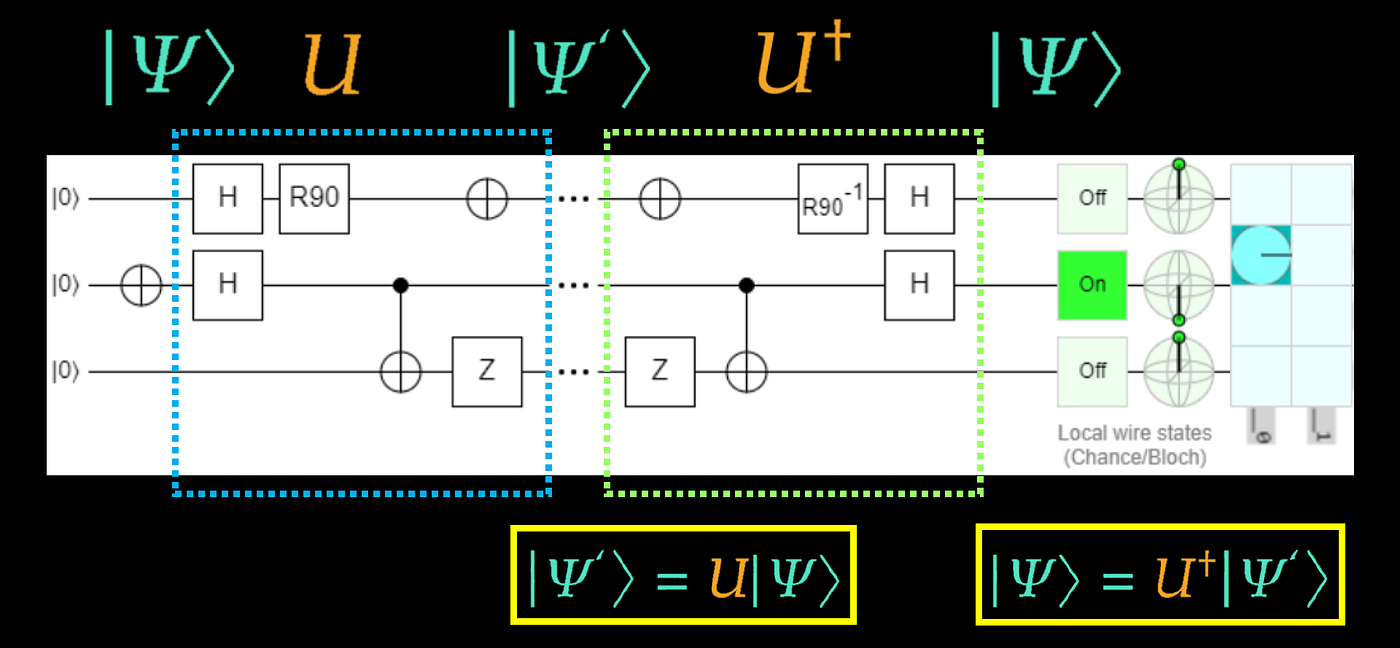Reversible operations in a quantum circuit. The output quantum state |Ψ’> is returned to |Ψ> after performing U conjugate transpose. The illustration is created by Quirk (Strilanc, 2019).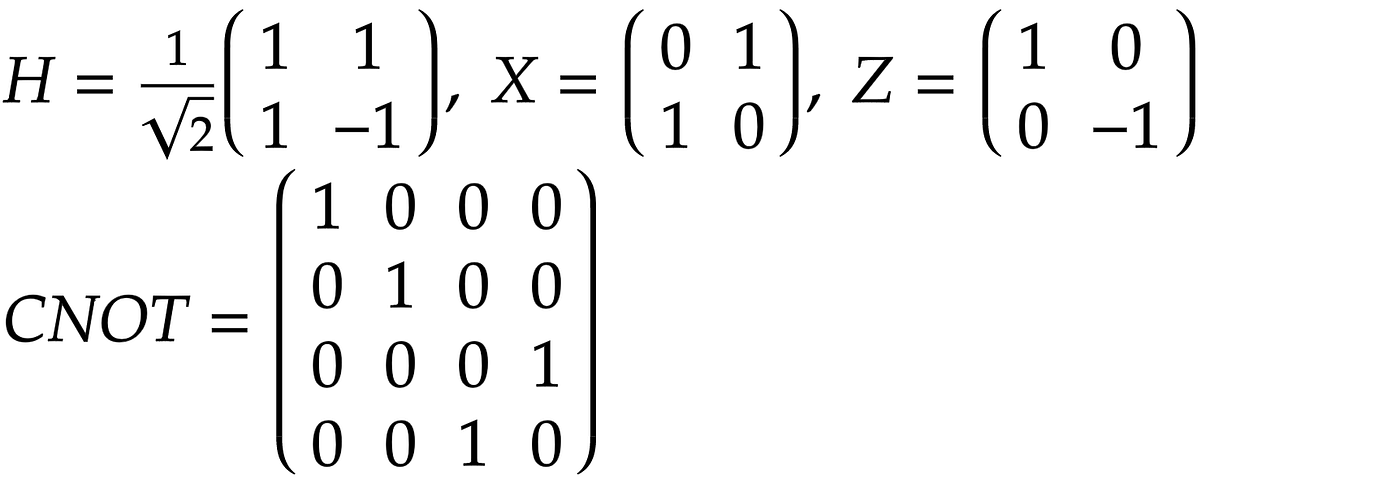Unitary matrices for H, X, Z, and CNOT gates.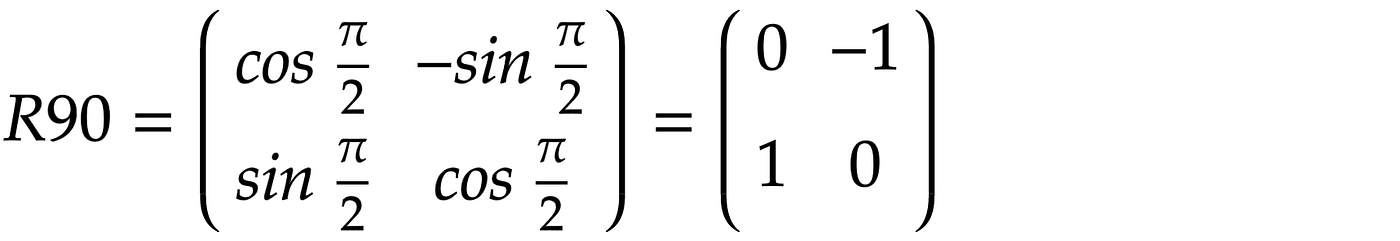Matrix for the R90 gate.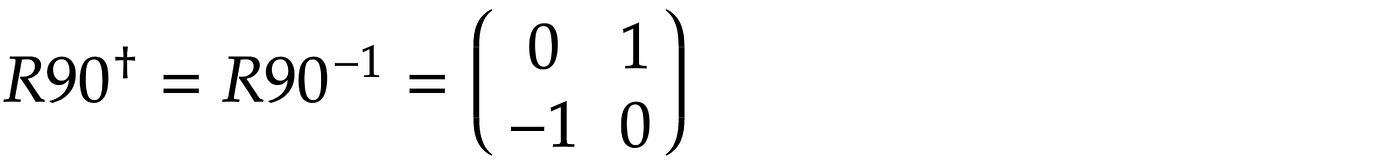Matrix for the R90-inverse gate.

# Executing the Quantum Circuit on IBM Quantum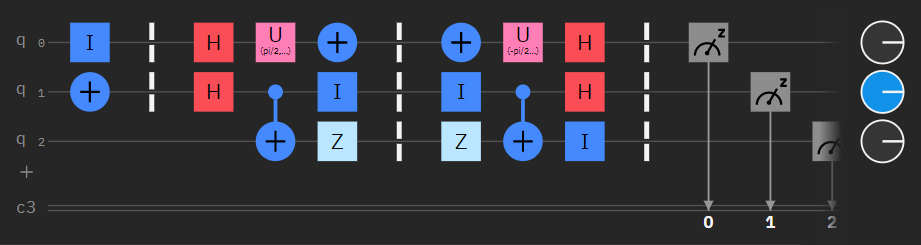The Reversible operations in a quantum circuit. The output quantum state |Ψ’> is returned to |Ψ> after performing U conjugate transpose. The illustration is created using IBM Quantum (IBM, 2021a).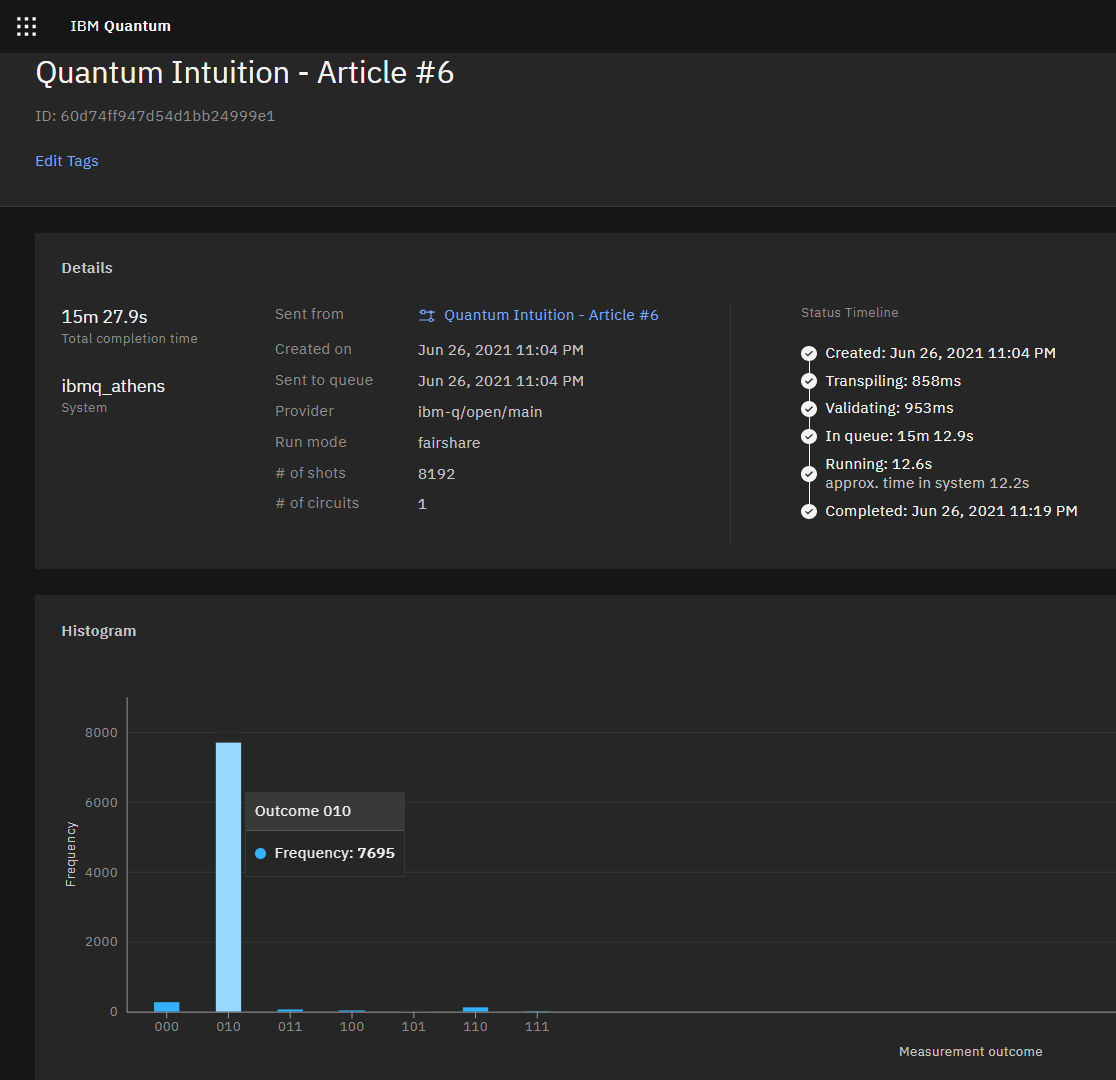The final result 010 after executing our quantum circuit with 8192 shots on the real quantum computer, IBM’s 5 qubits Quantum Computer “ibmq_athens.”Matrix for the R90 gate.
OPENQASM 2.0;include "qelib1.inc";qreg q;creg c;id q;x q;barrier q;barrier q;h q;h q;u(pi/2,-pi/2,pi/2) q;cx q,q;x q;id q;z q;barrier q;barrier q;barrier q;x q;id q;z q;u(-pi/2,-pi/2,pi/2) q;cx q,q;h q;h q;id q;barrier q;barrier q;barrier q;measure q -> c;measure q -> c;measure q -> c;
from qiskit import QuantumRegister, ClassicalRegister, QuantumCircuitfrom numpy import piqreg_q = QuantumRegister(3, 'q')creg_c = ClassicalRegister(3, 'c')circuit = QuantumCircuit(qreg_q, creg_c)circuit.id(qreg_q)circuit.x(qreg_q)circuit.barrier(qreg_q)circuit.barrier(qreg_q)circuit.h(qreg_q)circuit.h(qreg_q)circuit.u(pi/2, -pi/2, pi/2, qreg_q)circuit.cx(qreg_q, qreg_q)circuit.x(qreg_q)circuit.id(qreg_q)circuit.z(qreg_q)circuit.barrier(qreg_q)circuit.barrier(qreg_q)circuit.barrier(qreg_q)circuit.x(qreg_q)circuit.id(qreg_q)circuit.z(qreg_q)circuit.u(-pi/2, -pi/2, pi/2, qreg_q)circuit.cx(qreg_q, qreg_q)circuit.h(qreg_q)circuit.h(qreg_q)circuit.id(qreg_q)circuit.barrier(qreg_q)circuit.barrier(qreg_q)circuit.barrier(qreg_q)circuit.measure(qreg_q, creg_c)circuit.measure(qreg_q, creg_c)circuit.measure(qreg_q, creg_c)

--

--